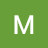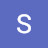Class 10 | Maths | Exercise 4.3 | ( Quadratic Equations ) | NCERT | CBSE | Maths Tutor |Class 10 | Maths | Exercise 4.3 | ( Quadratic Equations ) | NCERT | CBSE | Maths Tutor |

Class 10 | Ex -4.3 | Q7,8 and 9 | Math ( Quadratic Equations ) | NCERT | CBSE | Maths Tutor |

algebra tutors,Class 10 exercise 4.3,Class 10 maths,Class 10 quadratic equations,Class 10 maths Quadratic Equations,Class 10 ncert maths,Class 10 ncert,Class 10 cbse,Class 10 PSEB,Class 10 Exercise 4.3 Question no. 7,Class 10 Exercise 4.3 Question no. 8,Class 10 ex 4.3 q9,10th maths ncert exercise 4.3 q7,10th maths cbse exercise 4.3 q7,Maths tutor,10th maths exercise 4.3 ncert,10th maths ex. 4.3 cbse,10th maths ex. 4.3 pseb

algebratutors.org

1.Micky Uppal says:
2.Sonu Anand says: